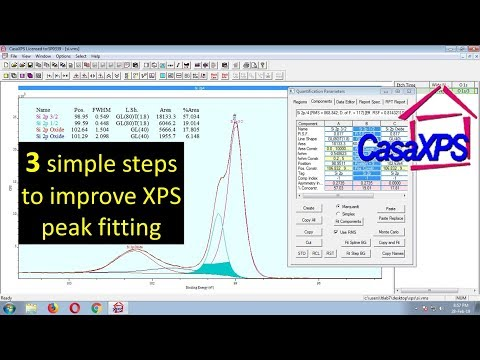# CasaXPS T3 - 3 Simple & Essential Steps to Improve XPS peak fittingI will show in this video, how you can improve, your XPS peak fitting,following a few simple steps.
I will use Si 2p as an example.
But, I will shows in a general way, so that, you can follow these steps for any element.
This is Si 2p narrow scan. If you can remember, in the prevous video,
I have shown about Si 2p. Identification, smoothening or noise reduction,
basic fitting etc. Now, Let's stat the fitting.
Create a region.
Si 2p. Here comes the first important step.
The back ground type. By default the background type is Shirley.
I want to see, how other functions will fit. I am changing Shirley to Silicon Tougaard.
To do that, I have to enter U Si. It seems not bad.
Let's check other functions similarly. Entering U SiO2 to use U SiO2 Tougaard.
This does not fit well. Next, Changing to, U Aluminium Tougaard,
Short form U Al. This looks okay. Let's see others too.
And the last one, U Polynomial Tougaard short form U Poly.
A very little change. Let's change back to Shirley.
I guess, Shirley works better. Keep this in mind,
Shirley will fit better in most of the cases. Because, RSF values stand good with Shirley.
So, in your second step, you should make sure, RSF values are correct.
The another factor which makes Shirley more usable than other, is that,
Shirley takes care about the asymmetry of the line shape near background.
So, our next step, which should be your third step, is Line shape.
There are two basic peak shapes: Gaussian and Lorentzian.
But, in the most of experimental cases, the shape is in between
the Gaussian, and the Lorentzian. Thus, the line shape is described by The Voigt function.
The Voigt function can be in two forms:
Product Form, and Sum Form. Additionally, the line shape can be asymmetric.
and exponential. While fitting, you need to know, at least
a few essential functions: GL(p) : Which is a Gaussian-Lorentzian product formula.
where, the mixing is determined by m = p/100.
For example, GL(100) is a pure Lorentzian, while GL(0) is pure Gaussian.
SGL(p) : Which is a Gaussian-Lorentzian sum formula,
where, the mixing is determined by m = p/100. For example, GL(100) is a pure Lorentzian
while GL(0) is pure Gaussian. GL(p)T(k) : Which is a Gaussian-Lorentzian
product formula, modified by the exponential blend.
SGL(p)T(k) : Which is a Gaussian-Lorentzian sum formula,
modified by the exponential blend.
A(a,b,n)GL(p): Which is a Gaussian-Lorentzian product formula,
modified by an asymmetric form. Examples are shown in this figure.
here a is 0.35, b is varied from 0.2 to 1, n is kept 0.
Considered as pure Gaussian, thus it is GL(0). Let's try these line shapes, one by one.
By looking the shape, we can assume, there are 3 peaks.
And, we know from the previous analysis, there could be
3 peaks: 2p 3/2, 2p 1/2 and one oxide peak.
Let's make it.
This is the first component. It's 2p 3/2.
Next, 2p 1/2. Last one, 2p oxide.
The position is not correct. Let' correct it. It must be here. 102.6.
Now, we can check, how the fit to data. Well, we can improve it.
Look at the envelope and the data. Try to find where are the gaps between those.
Here. And, here also.
We can increase the Gaussian contribution. Increasing 30 to 40.
Seems, better. Let's do it for other 2 also.
Then, fit these. looks better.
We can try increasing the Gaussian contribution more.
Let's use 60, this time. Better.
But, kind asymetry is observable, here. Let's try with asymmetry.
The function is A (0.35, 0.2, 0). Adding it to second one also.
Now,the gap reduced. What's about, if it is more of exponetial
bend, than the normal asymmetry function, what we
have used. We can check it.
Remove, asymmetry and add exponential bend. We can try with 1.5.
T(1.5) Now, both the 2p 3/2, and 2p 1/2 look fine.
But, the oxide peak is not so. Probably, there is one more peak here.
Let's add this. Now, checking the fitting.
This is great. Let's check entire region closely.
These two peaks can be a little more broader. Which means the Gaussian contribution.
Make it 80. Also, I will increase the exponential factor.
1.6. 1.7.
1.8.
Wow. this is better.
Maybe, this oxide peak, can be treated more. This left side is over estimated in the parameters.
We can decrease the Guassian contribution.
50.
40.
Let me know in your comment,
If you want to try or practice with the data file, which I have used.
I will stop here. See you in the next video.
Rietveld refinement in 4 minute │The simplest way to refine XRD results │ using MAUD Easiest & Best Way to do Rietveld Refinement Using FullProf software Suit Starting to end Multiple Peaks Fitting of XPS, Raman, PL spectroscopy │Curve Fitting using Origin How to Get data from image ( graph, results etc) │ Digitize your image │Easiest way XRD Analysis: Structure & Peak identification & indexing using JCPDS (PCPDFWIN) or COD databases Rietveld refinement with FullProf (T#2 ) │ How to Find Parameters to start refinement Easiest way to convert X ray diffraction (XRD) data file formats How to call a Phone without showing number │ Easiest way to make International Phone calls I Tried Wedding Dresses From Wish Full Face Using Only E.L.F. Makeup... I'm Speechless!